# Normalization of Neoclassical Fluid Equations

In this section, we shall estimate the relative sizes of the various terms appearing in our neoclassical fluid equations. Let us assume that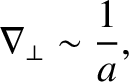(2.352)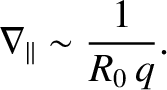(2.353)

As before, we shall adopt the drift ordering (2.113). It is helpful to define the following timescales: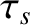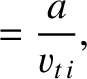(2.354)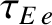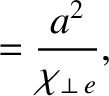(2.355)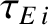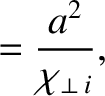(2.356)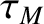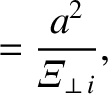(2.357)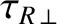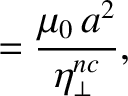(2.358)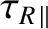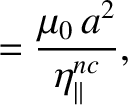(2.359)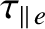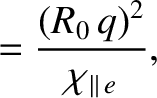(2.360)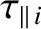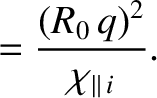(2.361)

Here,is the typical time required for a sound wave to traverse the plasma,is the typical time required for electron energy to diffuse out of the plasma,is the typical time required for ion energy to diffuse out of the plasma,is the typical time required for momentum to diffuse out of the plasma,is the typical time required for the perpendicular current to diffuse out of the plasma,is the typical time required for the parallel current to diffuse out of the plasma,is the typical time required for the electron temperature to attain equilibrium on magnetic flux-surfaces, andis the typical time required for the ion temperature to attain equilibrium on magnetic flux-surfaces. We shall assume that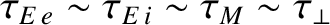. We shall also assume that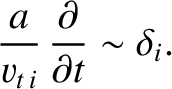(2.362)

The previous equation is an extension of the drift ordering, (2.113), that takes into account the fact that tearing modes usually propagate with respect to the MHD fluid at diamagnetic velocities .

It is helpful to define the following dimensionless parameters: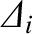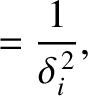(2.363)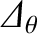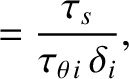(2.364)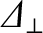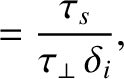(2.365)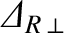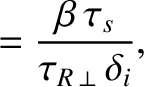(2.366)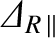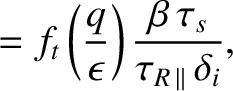(2.367)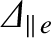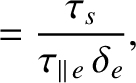(2.368)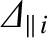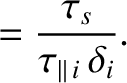(2.369)

Here,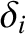is the ion magnetization parameter defined in Equation (2.110), whereas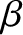is defined in Equation (1.23). Table 2.6 gives estimates for the dimensionless parameters defined in Equations (2.363)–(2.369) for a low-field and a high-field fusion reactor. As before, these estimates are made assuming that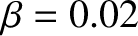,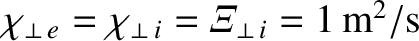, and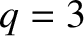.

Table: 2.6 Dimensionless parameters used in the normalization of the neoclassical fluid equations in a low-field and a high-field tokamak reactor. See Equations (2.363)–(2.374).
 Low-Field High-Field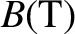5.0 12.0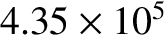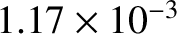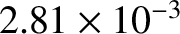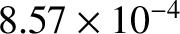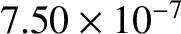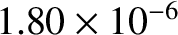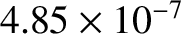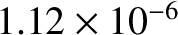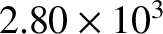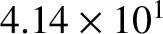Our neoclassical fluid equations, (2.326), (2.331), and (2.338)–(2.340), can be written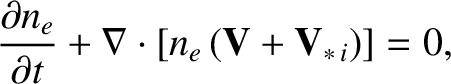(2.370)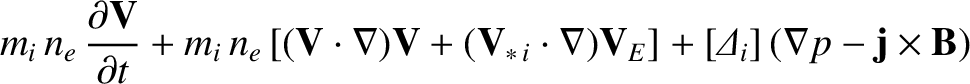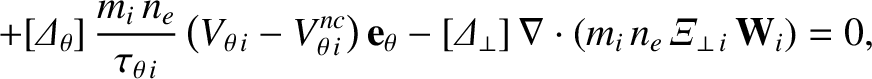(2.371)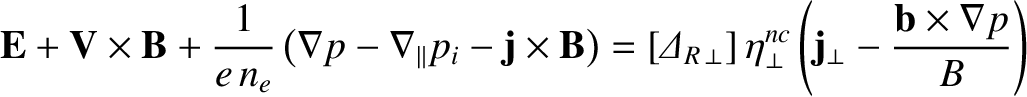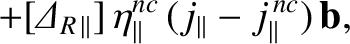(2.372)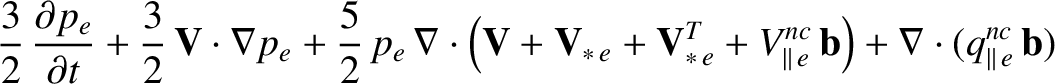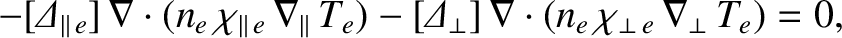(2.373)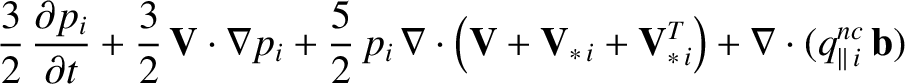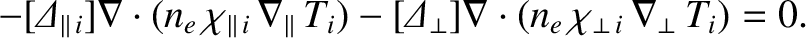(2.374)

Here, the factors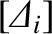,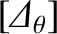,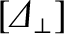, et cetera, indicate that the terms they precede are larger or smaller than terms preceded by no factor by the dimensionless parameter contained within the square brackets.

According to Table 2.6, the dominant parallel diffusivity term in the electron energy conservation equation, (2.373), yields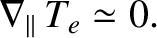(2.375)

In other words, the parallel electron energy diffusivity in a tokamak fusion reactor is sufficiently large to ensure that the electron temperature is uniform on magnetic flux-surfaces. Likewise, according to Table 2.6, the dominant parallel diffusivity term in the ion energy conservation equation, (2.374), gives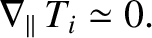(2.376)

In other words, the parallel ion energy diffusivity in a tokamak fusion reactor is sufficiently large to ensure that the ion temperature is uniform on magnetic flux-surfaces. According to Table 2.6, the dominant terms in the plasma equation of motion, (2.371), yield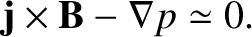(2.377)

In other words, the plasma in a tokamak fusion reactor exists in a state of approximate force balance. The previous equation suggests that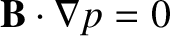. When combined with Equations (2.375) and (2.376), this relation gives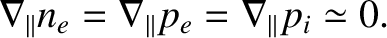(2.378)

We conclude that the electron number density, the electron pressure, and the ion pressure are all uniform on magnetic flux-surfaces in a tokamak fusion reactor. According to Table 2.6, the dominant terms in the plasma Ohm's law, (2.372), yield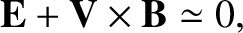(2.379)

where use has been made of Equations (2.377) and (2.378). Thus, we conclude that the plasma in a tokamak fusion reactor satisfies the so-called perfect conductivity or flux-freezing constraint. As is well known, this constraint forbids any change in the topology of magnetic field-lines . Finally, according to Table 2.6, the dominant term in the electron number density continuity equation, (2.370), gives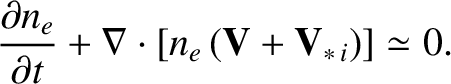(2.380)

Equations (2.375)–(2.380) are known collectively as the equations of marginally-stable ideal-MHD .